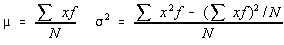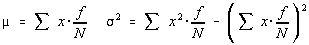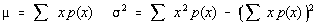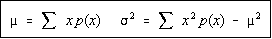Probability Distributions

Probability Functions

A probability function is a function which assigns probabilities to the values of a random variable.

• All the probabilities must be between 0 and 1 inclusive
• The sum of the probabilities of the outcomes must be 1.

If these two conditions aren't met, then the function isn't a probability function. There is no requirement that the values of the random variable only be between 0 and 1, only that the probabilities be between 0 and 1.

Probability Distributions

A listing of all the values the random variable can assume with their corresponding probabilities make a probability distribution.

A note about random variables. A random variable does not mean that the values can be anything (a random number). Random variables have a well defined set of outcomes and well defined probabilities for the occurrence of each outcome. The random refers to the fact that the outcomes happen by chance -- that is, you don't know which outcome will occur next.

Here's an example probability distribution that results from the rolling of a single fair die.
 x 1 2 3 4 5 6 sum p(x) 1/6 1/6 1/6 1/6 1/6 1/6 6/6=1

Mean, Variance, and Standard Deviation

Consider the following.

The definitions for population mean and variance used with an ungrouped frequency distribution were:Some of you might be confused by only dividing by N. Recall that this is the population variance, the sample variance, which was the unbiased estimator for the population variance was when it was divided by n-1.

Using algebra, this is equivalent to:Recall that a probability is a long term relative frequency. So every f/N can be replaced by p(x). This simplifies to be:What's even better, is that the last portion of the variance is the mean squared. So, the two formulas that we will be using are:Here's the example we were working on earlier.
 x 1 2 3 4 5 6 sum p(x) 1/6 1/6 1/6 1/6 1/6 1/6 6/6 = 1 x p(x) 1/6 2/6 3/6 4/6 5/6 6/6 21/6 = 3.5 x^2 p(x) 1/6 4/6 9/6 16/6 25/6 36/6 91/6 = 15.1667

The mean is 7/2 or 3.5
The variance is 91/6 - (7/2)^2 = 35/12 = 2.916666...
The standard deviation is the square root of the variance = 1.7078

Do not use rounded off values in the intermediate calculations. Only round off the final answer.

You can learn how to find the mean and variance of a probability distribution using lists with the TI-82 or using the program called PDIST.